Security Market Indexes | IFT World
IFT Notes for Level I CFA® Program
IFT Notes for Level I CFA® Program

# Part 2

Equal Weighted Index

The equal weighting method assigns an equal weight to each constituent security at inception.

An equal weighted index can be created by allocating an equal amount of money to all securities.

Let’s say, you have $180,000 to invest. You will invest$60,000 each in shares of A, B, and C trading at $4,$6 and $10 respectively. This would mean 15,000 shares of A, 10,000 shares of B and 6,000 shares of C. However, at the end of the period, the index will no longer be equally weighted as share prices may have changed. So, it requires rebalancing (buy shares of depreciated stock, sell shares of appreciated stock) for the index to be equal weighted. The return of an equal weighted index is calculated as a simple average of the returns of the index stocks.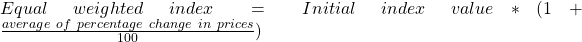Example Given the following data, compute the price return and total return.  Beginning of period Price End of period Price Dividend/share A 4 2 0 B 6 6 1 C 10 14 2 Solution: Price return for A: -50%; B: 0%; C: 40%. Since weights are equal, price return =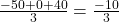= -3.3%. Dividend return for A: 0%; B: 16.67%; C: 20%. Total dividend return =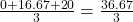= 12.22%. Total return = Price return + Dividend return = -3.3 + 12.22 = 8.9%. Advantage of equal weighting: Simplicity. Limitations of equal weighting: • Securities with largest market value are underrepresented; those with lowest market value are overrepresented. • Maintaining equal weights requires frequent rebalancing. If not rebalanced periodically, the chances of drifting away from the weights are high. Market Capitalization Weighted Index In this method, weight of each security is determined by dividing its market capitalization with total market capitalization.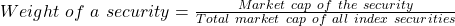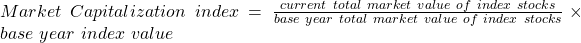Example The following data is given:  Shares outstanding Beginning of period price End of period price Dividends per share A 500 4 2 0 B 100 6 6 1 C 100 10 14 2 1. Given the data, what divisor must be used such that the initial index value is 1,000? 2. Compute the: 1) final index value 2) price return and total return. 3. Compute the price return if stock C has a market float of 40%. Solution: 1. Sum of market capitalization of all securities = 500 x 4 + 100 x 6 + 100 x 10 = 3,600 Initial index value = 1,000 =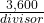= 3.6.Anytime in the future to calculate the index value, this value of the divisor is used. 1. The weights of the three securities are tabulated below:  Price return Market capitalization weights A (2 – 4) / 4 = – 0.5 2,000 / 3,600 = 0.56 B (6 – 6) / 6 = 0 600 / 3,600 = 0.17 C (14 – 10) / 10 = 0.4 1,000 / 3,600 = 0.28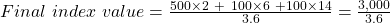= 833.33. Price return =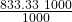= -16.67%. Price return can also be calculated as: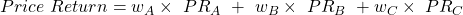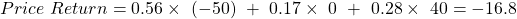Dividend return =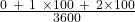= 8.3%. Total return = -16.67 + 8.3 = ~ -8.3%. 1. Assume the remaining 60% of stock C is not available for trading as the founding family owns them. Only 40% of shares are available for trading. To calculate the price return, instead of using 100%, only 40% of shares are used in calculation. In this case, 40 shares. Sum of market capitalization of all securities = 500 x 4 + 100 x 6 + 40 x 10 = 3,000 Initial index value = 1,000 =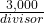; divisor = 3. Final index value =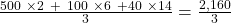= 720. Price return =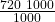= -28%. A float-adjusted market-capitalization weighted index weights each of its constituent securities by price and the number of its shares available for public trading, i.e. by excluding the shares held by the promoter group etc. Advantages of market capitalization weightingConstituent securities are correctly represented in proportion to their value in the market. Limitations of market capitalization weighting: Securities whose prices have risen or fallen the most see a big change in their weights. Stocks whose prices have increased are over weighted; similarly, stocks whose prices have fallen are underweighted. Fundamental Weighted Index Fundamental weighting addresses the disadvantages of using market capitalization as weights. Instead of using a stock’s price as a measure, fundamental weighting uses measures such as book value, cash flow, revenue, earnings and dividends to calculate the weight of each security. For instance, a stock with higher earnings yield (earnings/price) than the overall market will have more weight in a fundamental-weighted index than in a market-weighted index. This weighting method is biased towards value stocks. This is sometimes called a ‘value tilt’ and is illustrated in the example below. Example Compute the price return for the following index. Weight the securities based on earnings.  Shares outstanding (in million) Beginning of period price price Earning (in$ million) End of period price A 500 4 20 2 B 100 6 20 6 C 100 10 20 14

Solution:

All the three companies have earnings of $20 million and total earnings of$60 million.

Earnings yield, earnings weight and price return of the three companies:

 Earnings yield Earnings weight Price return A 20 / (500 x 4) = 1% 20 / 60 = 33.3% (2 – 4) / 4 = -0.5 B 20 / (100 x 6) = 3.3% 20 / 60 = 33.3% (6 – 6) / 6 = 0 C 20 / (100 x 10) = 2% 20 / 60 = 33.3% (14 – 10) / 10 = 0.4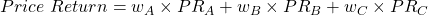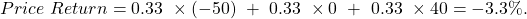All the three securities have equal weights here as the earnings are equal. Under the market capitalization method, A would have highest weight and B would have the lowest weight. In other words, a value stock like B (low P/E ratio or high earnings yield) has more weightage in the fundamental-weighted method than it would have in the market capitalization method.

Summary of Results

The table below compares all the weighting methods.

 Number of shares BOP price EOP Price Earnings Dividends/share A 500 4 2 20 0 B 100 6 6 20 1 C 100 10 14 20 2

 Method Price Return Total Return Price 10% 25% Equal -3.3% 8.9% Market Cap -16.7% -8.3% Fundamental -3.3% 8.9%

The pros and cons of the different index weighting methods are shown below.

 Method Pros Cons Price Simple Arbitrary weights. Equal Simple High market cap stocks are under-represented. Requires frequent rebalancing. Market Cap Securities held in proportion to their value Influenced by overpriced securities. Fundamental Value tilt Does not consider market value. Requires rebalancing.

### 4.1 Rebalancing

Rebalancing means adjusting the weights of constituent securities in an index to maintain the weight of each security in the index. The weights do not remain constant as the prices of securities change. For weighting methods like price-weighted and market-weighted index, rebalancing is not necessary as the weight is determined by the price. However, as we saw in the case of equal-weighting method, the weights digress heavily when the price of a security appreciates/depreciates. If rebalancing happens too often, then the transaction costs will be high. If rebalancing does not happen often enough, then the portfolio will digress from equal weights.

### 4.2 Reconstitution

Reconstitution is the process of changing the constituent securities in an index. It is part of the rebalancing cycle. The frequency of reconstitution varies from index to index. When a constituent security no longer meets the necessary criteria it is removed from the index and a new security is added. For example, a stock might be part of a large-cap index but after an erosion of over 80% of its market cap it no longer meets the large cap criteria. This stock will be removed from the index and another one which meets the criteria will be added.

LIVE WEEKEND CLASSES *For 2024 Exam candidates*
This is default text for notification bar